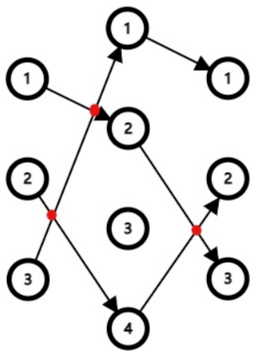# #674. 【NOI2021】路径交点

$$(P_j-Q_j) \times (P_{j+1}-Q_{j+1}) < 0$$### 样例一

#### input

1
3
2 3 2
4 4
1 1
1 2
2 1
2 3
1 2
2 1
3 1
3 2



#### output

1



#### explanation

$1$ $(1, 1, 2)$ $(2, 3, 1)$ $1$
$2$ $(1, 2, 1)$ $(2, 1, 2)$ $2$
$3$ $(1, 2, 1)$ $(2, 3, 2)$ $0$

### 数据范围

$1 \sim 4$ $2$ $10$
$5 \sim 6$ $10$ A,B
$7 \sim 8$ A
$9 \sim 10$
$11 \sim 13$ $2$ $100$
$14 \sim 15$ $100$ A,B
$16 \sim 17$ A
$18 \sim 20$